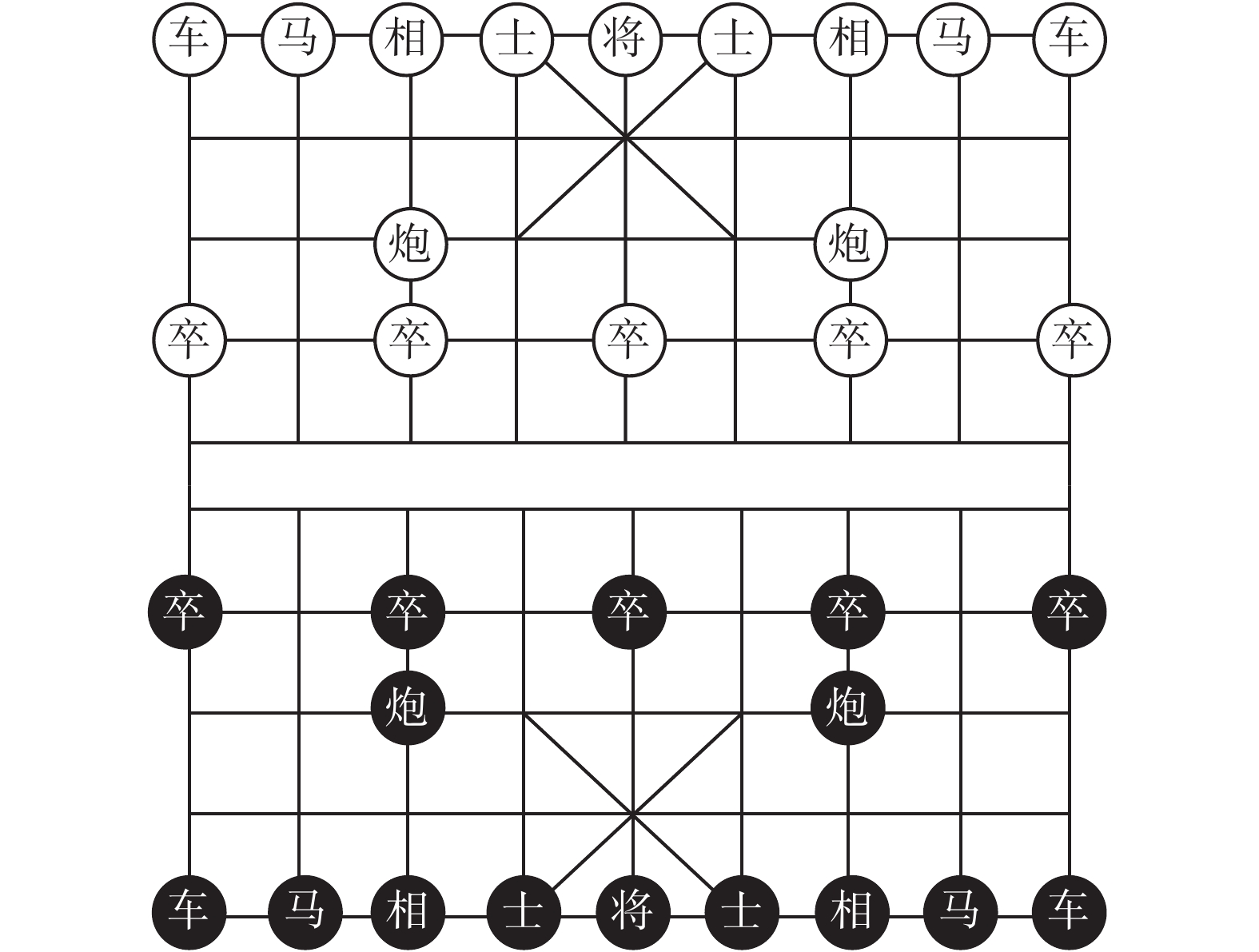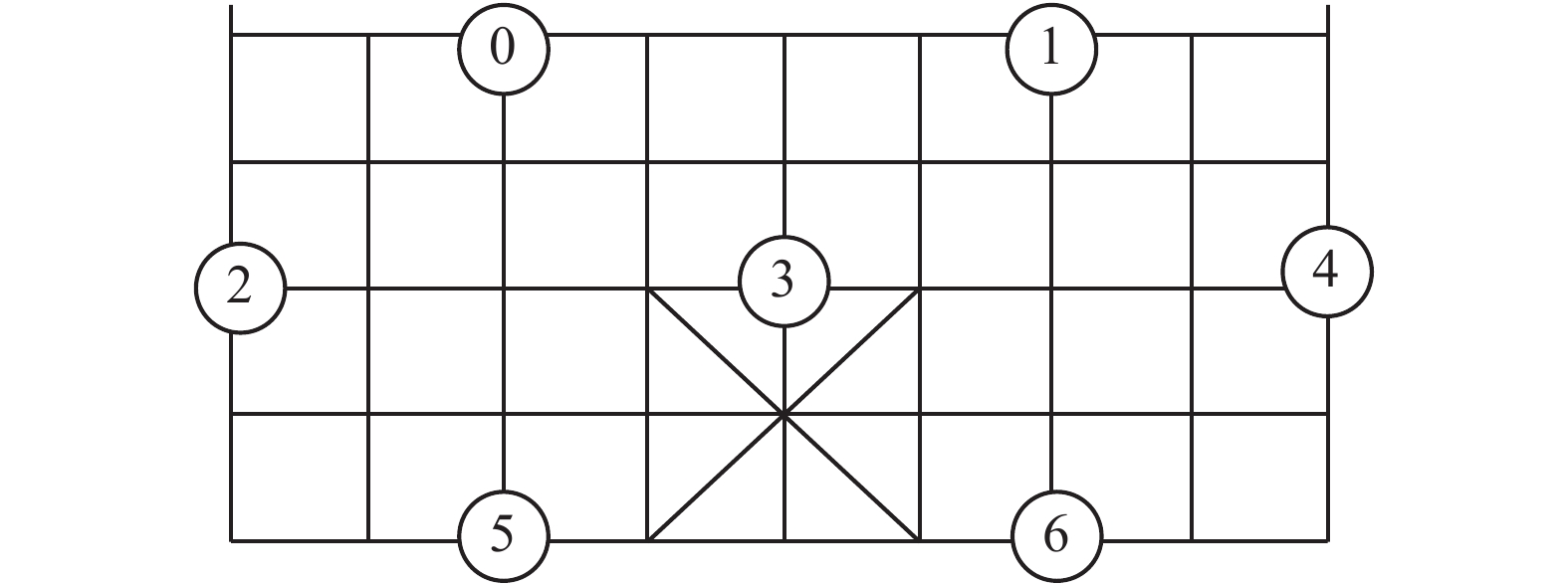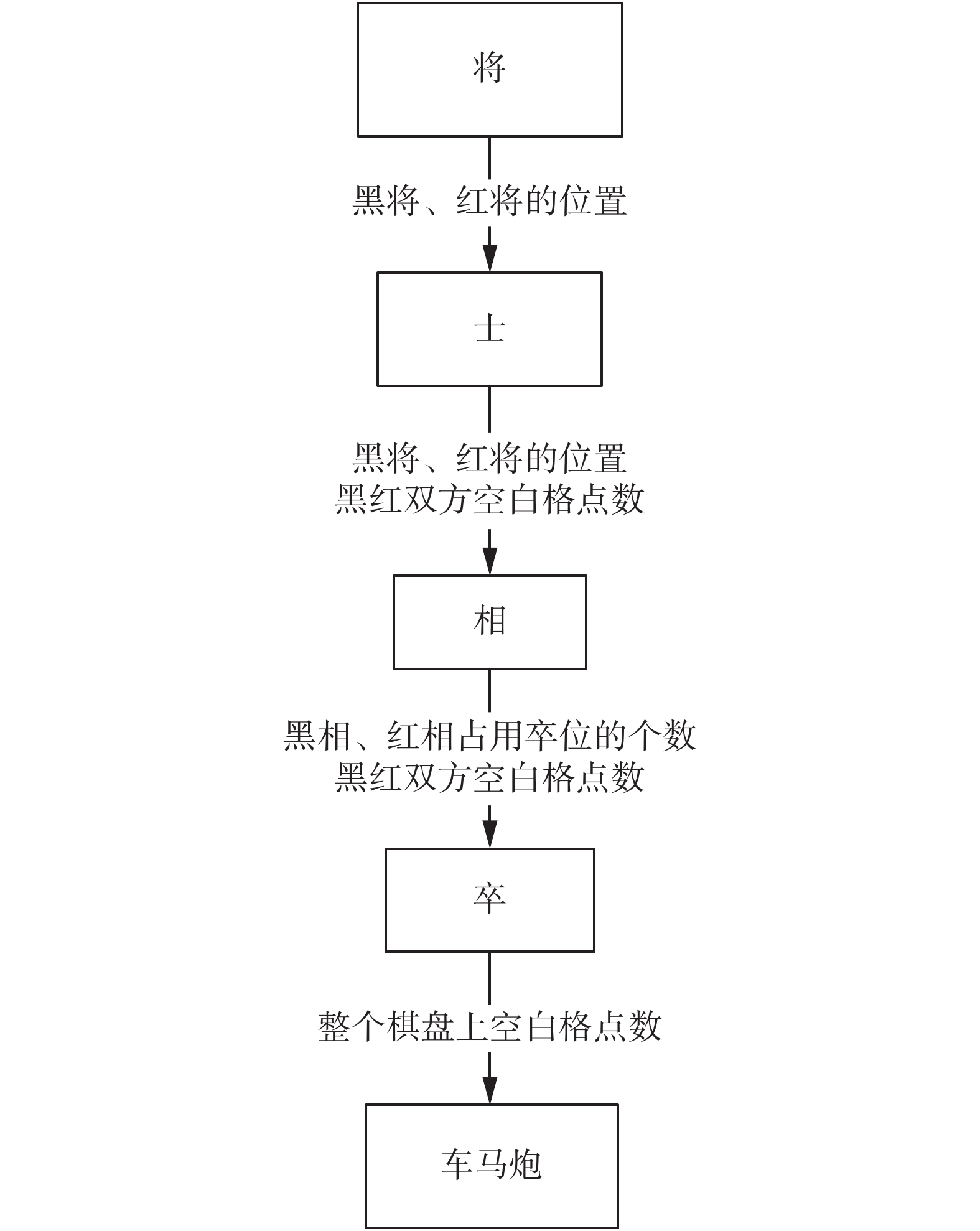﻿ 动态规划求解中国象棋状态总数
«上一篇文章快速检索 高级检索

 智能系统学报2019, Vol. 14Issue (1): 108-114  DOI: 10.11992/tis.2018030080

### 引用本文WEI Yinfu, LI Zhoujun. A method for calculating the total number of states of Chinese chess on the basis of dynamic programming[J]. CAAI Transactions on Intelligent Systems, 2019, 14(1): 108-114. DOI: 10.11992/tis.201803008.### 文章历史

A method for calculating the total number of states of Chinese chess on the basis of dynamic programming
WEI Yinfu, LI Zhoujun
The Institute of Intelligent Information Processing, School of Computer Science and Engineering, Beihang University, Beijing 100191, China
Abstract: The space complexity of Chinese chess is a primary index for analyzing the complexity of Chinese chess, which is a counting problem of calculating the number of states of Chinese chess. Given the features of Chinese chess, this problem can be divided into several subproblems that can be solved by dynamic programming to obtain a precise solution of the total number of states of Chinese chess. Our results show that the total number of states of Chinese chess mentioned in previous papers is inaccurate and much higher than the actual number of states (1039.88). Finally, the main idea of the counting method was summarized based on dynamic programming, and illustrations for some uses of the method were provided.
Key words: computer games    Chinese chess    combinatorial counting    space complexity    dynamic programming    counting method    problem solving    state space

1 问题描述 1.1 中国象棋棋盘棋子Download: 图 1 中国象棋开始局面 Fig. 1 The start state of Chinese Chess表 1 棋子英文表示及其缩写 Tab.1 The chess representation and their abbreviation
1.2 走子规则Download: 图 2 相的可去位置及其编号 Fig. 2 Xiang’s positions and numberDownload: 图 3 九宫格内位置编号 Fig. 3 Jiugong position and number

1.3 问题定义

 $S = \mathop \sum \limits_{{\rm{ChessType}} \in {\text{全部棋型}}} {\rm{put}}\left( {{\rm{ChessType}}} \right)$ (1)

1) 本文讨论的是中国象棋可能出现的全部状态，在每步着法都完美的情况下，从初始状态出发可能无法到达全部状态。

2) 本文统计的状态集合中每个状态双方“将”都是存在的，即本文计算的全部局面都是游戏尚未结束的局面。

3) 本文讨论的状态包括“将帅见面”的情况。在中国象棋规则中，促成“将帅见面”局面的一方为输。

2 问题求解 2.1 摆放次序

1) 当车占用0个相位时，车有83个位置可选，相有7个位置可选，这种情况有 $C_{83}^2 \times C_7^2$ 种局面。

2) 当车占用1个相位时，第1个车有7种相位可选，第2个车有83个位置可选，2个相有6个位置可选，这种情况有 $C_7^1 \times C_{83}^1 \times C_6^2$ 种局面。

3) 当车占用2个相位时，摆放方式有 $C_7^2 \times$ $C_5^2$ 种。

2.2 问题分解

2.2.1 将

1) s = 0；

2) 对于红将的每个位置KR∈[0, 9)；

3) 对于黑将的每个位置KB∈[0, 9)；

4) s+=getShi(KR, KB)

 $S = \mathop \sum \limits_{{K_R} = 0}^8 \mathop \sum \limits_{{K_B} = 0}^8 {\rm{getShi}}\left( {{K_R},{K_B}} \right)$ (2)
2.2.2 士

1) s=0；

2) 定义红士可去位置个数：若KR不在士位上NR=5，否则NR=4；

3) 定义黑士可去位置个数：若KB不在士位上NB=5，否则NB=4；

4) 对于红士个数的可取值AR∈{0, 1, 2}；

5) 对于黑士个数的可取值AB∈{0, 1, 2}；

6) 红方空白格点数SpaceR=45−1−AR

7) 黑方空白格点数SpaceB=45−1−AB

8) s+= $C_{{N_R}}^{{A_R}}C_{{N_B}}^{{A_B}}$ getXiang(KR, KB, SpaceR, SpaceB)

2.2.3 相

1) “将”可能会占用相位，影响“相”的可摆放位置的个数；

2) 红方和黑方各自的空白格点数，影响“卒车马炮”的摆放。

“相卒车马炮”的摆法只跟4个变量有关：红将位置、黑将位置、红方空白格点数、黑方空白格点数。这4个变量一旦确定，“相卒车马炮”的摆法个数便随之确定。求解子问题“相卒车马炮”需要先考虑相的摆法。根据“将”是否已经放在如图3的1号位置，可以确定相的可选位置的个数。

1) 定义红相可去位置个数：若KR不在相位上NR=7否则NR=6；

2) 定义黑相可去位置个数：若KB不在相位上NB=7否则NB=6；

3) s=0；

4) 对于红相个数的可取值BR∈{0, 1, 2}；

5) 对于黑相个数的可取值BB∈{0, 1, 2}；

6) 对于红相占用红卒位置的个数BPR∈{0, 1, 2}；

7) 对于黑相占用黑卒位置的个数BPB∈{0, 1, 2}；

8) 相的放法种数 ${\rm{placeXiang}} = C_2^{B{P_R}}C_2^{B{P_B}}C_{{N_R} - 2}^{{B_R} - B{P_R}}$ $C_{{N_B} - 2}^{{B_B} - B{P_B}}$

9) s+=placeXiang×getZu(BPR, BPB, SpaceRBR, SpaceBBB)。

2.2.4 卒

“卒”需要分为2类进行讨论：过河卒和未过河卒。各方过河卒个数加上未过河卒的个数不超过5，需考虑红方、黑方各自的过河卒、未过河卒共4类棋子的摆放。

1) SpaceB表示黑方棋盘空白格点数，SpaceR表示红方棋盘空白格点数；

2) PR表示红方未过河卒的个数，PB表示黑方未过河卒的个数；

3) $P_R'$ 表示红方过河卒的个数， $P_B'$ 表示黑方过河卒的个数。

 $\mathop \sum \limits_{i = 0}^{\min \left( {BP,P} \right)} {2^{P - i}}C_{5 - BP}^{P - i}$ (3)

2.2.5 车马炮

“车马炮”的摆放仅与一个变量有关，即整个棋盘上空白格点数。枚举红黑双方车、马、炮的个数，使用分步乘法计算有多少种摆法，如式(4)：

 $\mathop \sum \limits_{{R_B} = 0}^2 \mathop \sum \limits_{{R_R} = 0}^2 \mathop \sum \limits_{{H_B} = 0}^2 \mathop \sum \limits_{{H_R} = 0}^2 \mathop \sum \limits_{{C_B} = 0}^2 \mathop \sum \limits_{{C_R} = 0}^2 {\rm{jvmapao}}\left( {{R_B},{R_R},{H_B},{H_R},{C_B},{C_R}} \right)$ (4)

jvmapao(RB, RR, HB, HR, CB, CR)函数可以使用分步乘法实现。例如，摆完“将相士卒”之后剩余80个空白格点，在这些空白位置上摆放2个红车、2个红马、2个黑炮。根据分步乘法很容易得出摆法总数为 $C_{80}^2 \times C_{78}^2 \times C_{76}^2$ 。jvmapao函数实现如以下伪代码所示：

1) $s = C_{{\rm{Space}}}^{{R_B}} \times C_{{\rm{Space}} - {R_B}}^{{R_R}}$

2) Space=Space−RBRR

3) $s = s \times C_{{\rm{Space}}}^{{H_B}} \times C_{{\rm{Space}} - {H_B}}^{{H_R}}$

4) Space=Space−HBHR

5) $s = s \times C_{{\rm{Space}}}^{{C_B}} \times C_{{\rm{Space}} - {C_B}}^{{C_R}}$

2.3 动态规划方法加速求解

1) 将的摆放；

2) 士的摆放，输入为红将、黑将的位置；

3) 相的摆放，输入为红将、黑将的位置、红黑双方空白格点数4个变量；

4) 卒的摆放，输入为红黑双方空白格点数、红黑双方相占用卒位的个数；

5) 车马炮的摆放，输入为整个棋盘上空白格点数。Download: 图 4 各个部分的输入参数 Fig. 4 The input of every block

3 实验结果与验证表 2 参考文献中中国象棋空间复杂度 Tab.2 The Space Complexity of the Reference Document

4 结论和展望

  王晓鹏, 王骄, 徐心和, 等. 中国象棋与国际象棋比较分析[J]. 重庆工学院学报, 2007, 21(1): 71-76. WANG Xiaopeng, WANG Jiao, XU Xinhe, et al. A comparative analysis between chess and Chinese chess[J]. Journal of Chongqing institute of technology, 2007, 21(1): 71-76. DOI:10.3969/j.issn.1674-8425-B.2007.01.018 (0)  徐心和, 郑新颖. 棋牌游戏与事件对策[J]. 控制与决策, 2007, 22(7): 787-790. XU Xinhe, ZHENG Xinying. Card and board games and event game theory[J]. Control and decision, 2007, 22(7): 787-790. DOI:10.3321/j.issn:1001-0920.2007.07.014 (0)  SILVER D, HUANG A, MADDISON C J, et al. Mastering the game of Go with deep neural networks and tree search[J]. Nature, 2016, 529(7587): 484-489. DOI:10.1038/nature16961 (0)  SILVER D, SCHRITTWIESER J, SIMONYAN K, et al. Mastering the game of Go without human knowledge[J]. Nature, 2017, 550(7676): 354-359. DOI:10.1038/nature24270 (0)  SILVER D, HUBERT T, SCHRITTWIESER J, et al. Mastering chess and shogi by self-play with a general reinforcement learning algorithm[J]. arXiv: 1712.01815, 2017. (0)  高强, 郭琛. 哈希技术在中国象棋机器博弈系统中的应用研究[J]. 科学技术与工程, 2008, 8(17): 4869-4872. GAO Qiang, GUO Chen. Technology of hashing and its application research in hybrid game tree search engine of Chinese chess[J]. Science technology and engineering, 2008, 8(17): 4869-4872. DOI:10.3969/j.issn.1671-1815.2008.17.022 (0)  王骄, 徐长明, 徐心和. 中国象棋计算机博弈残局处理系统[C]//2006中国机器博弈学术研讨会. 北京, 中国, 2006. WANG Jiao, XU Changming, XU Xinhe. Endgame system in computer Chinese chess[C]//2006 Chinese Computer Games Seminar. Beijing, China, 2006. (0)  吴丽贤, 和力. 一种中国象棋残局棋谱自动生成算法[J]. 云南民族大学学报(自然科学版), 2010, 19(6): 435-438. WU Lixian, HE Li. An automatic generation algorithm for the manual of Chinese chess endgames[J]. Journal of Yunnan university of nationalities (natural sciences edition), 2010, 19(6): 435-438. (0)  马麟. 关于中国象棋的发展论述[J]. 求知导刊, 2016(2): 31. MA Lin. The develop of Chinese chess[J]. Journal of seeking knowledge guide, 2016(2): 31. DOI:10.3969/j.issn.2095-624X.2016.02.022 (0)  黄晨. 棋类游戏中的先行权[J]. 智能系统学报, 2007, 2(3): 91-94. HUANG Chen. The first-move advantage in board games[J]. CAAI transactions on intelligent systems, 2007, 2(3): 91-94. (0)  王玉霞. 组合计数中的映射原理及应用[J]. 喀什师范学院学报, 2005, 26(3): 31-32. WANG Yuxia. Mapping principle of combinational counting[J]. Journal of Kashgar teachers college, 2005, 26(3): 31-32. DOI:10.3969/j.issn.1006-432X.2005.03.012 (0)  胡冠章. 组合计数的群论与计算机方法[J]. 数学进展, 1997, 26(1): 1-12. HU Guanzhang. Group theory and computer methods for combinatorial enumerations[J]. Advances in mathematics, 1997, 26(1): 1-12. DOI:10.11845/sxjz.1997.26.01.1 (0)  曹博瑞, 刘铁. 映射法研究组合计数问题[J]. 文理导航, 2017(11): 4. CAO Borui, LIU Tie. Solve combinational counting problem by mapping[J]. Guiding on art and science, 2017(11): 4. (0)  王跃进. 映射观点下一些组合问题及其计数公式[J]. 上海中学数学, 2007(11): 40-41. WANG Yuejin. Several combinational counting problem based on mapping[J]. Middle school math of Shanghai, 2007(11): 40-41. DOI:10.3969/j.issn.1672-7495.2007.11.021 (0)  高见元. 动态规划算法的运用方法探讨[J]. 软件导刊, 2007(10): 136-138. GAO Jianyuan. Research on dynamic programming algorithm[J]. Software guide, 2007(10): 136-138. (0)  宛楠, 张义. 动态规划算法分析[J]. 长江大学学报(自然科学版), 2013, 10(7): 34-36. WAN Nan, ZHANG Yi. The analysis of dynamic algorithm[J]. Journal of Yangtze university (natural science edition), 2013, 10(7): 34-36. (0)  徐付霞. 大型动态规划的分解算法[J]. 唐山师专学报, 1998, 22(5): 6-9. XU Fuxia. Decomposable algorithm for large dynamic programming[J]. Journal of Tangshan normal university, 1998, 22(5): 6-9. (0)  王军祥. 动态规划算法原理及应用研究[J]. 电脑知识与技术, 2006(36): 150-151. WANG Junxiang. Research on the principle and application of dynamic programming algorithm[J]. Computer knowledge and technology, 2006(36): 150-151. (0)  ALLIS L V. Searching for solutions in games and artificial intelligence[D]. The Netherlands: University of Limburg, 1994. http://cn.bing.com/academic/profile?id=b1b876953627df69dbb12d92d0a5dfce&encoded=0&v=paper_preview&mkt=zh-cn (0)  涂志坚. 电脑象棋的设计与实现[D]. 广州: 中山大学, 2004. TU Zhijian. Design and implementation of computer Xiangqi[D]. Guangzhou: Sun Yat-sen University, 2004. (0)  徐心和, 王骄. 中国象棋计算机博弈关键技术分析[J]. 小型微型计算机系统, 2006, 27(6): 961-969. XU Xinhe, WANG Jiao. Key technologies analysis of Chinese chess computer game[J]. Mini-micro systems, 2006, 27(6): 961-969. DOI:10.3969/j.issn.1000-1220.2006.06.001 (0)